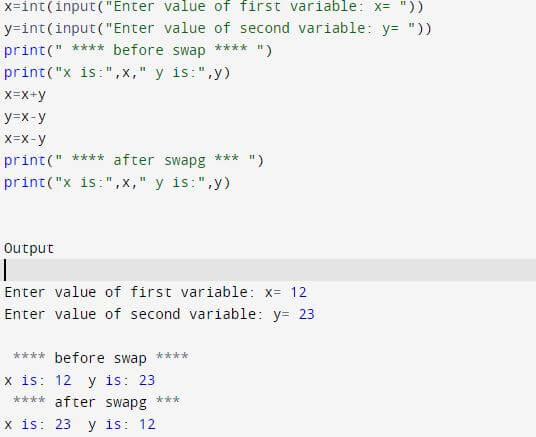# Python Program To Swap Two Numbers without Temporary Variable

Published January 07, 2021

In This Python program we will learn swap two numbers without using temporary variable.

Program Steps:

• Take two numbers from the user and save them in two different variables
• Add both numbers and store the value in first number
• Substract second value from the first variable value and store it in second variable
• Now, subtract first value from the second variable and store it in first variable.
• Now print the values.Program to swap two numbers

 x=int(input("Enter value of first variable: x= ")) y=int(input("Enter value of second variable: y= ")) print(" **** before swap **** ") print("x is:",x," y is:",y) x=x+y y=x-y x=x-y print(" **** after swap *** ") print("x is:",x," y is:",y)

Output

 Enter value of first variable: x= 102 Enter value of second variable: y= 123 **** before swap ****  x is: 102  y is: 123  **** after swap ***  x is: 123  y is: 102

Conclusion: This way we can swap numbers without using temporary variable in python.

 Article Contributed By :1520 Views
Projects By category
Categories